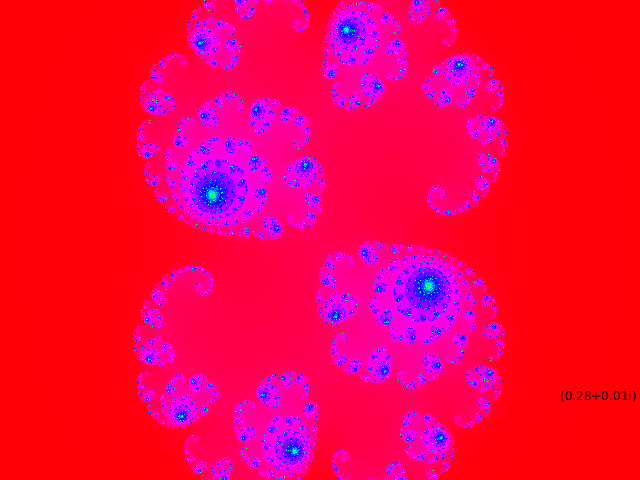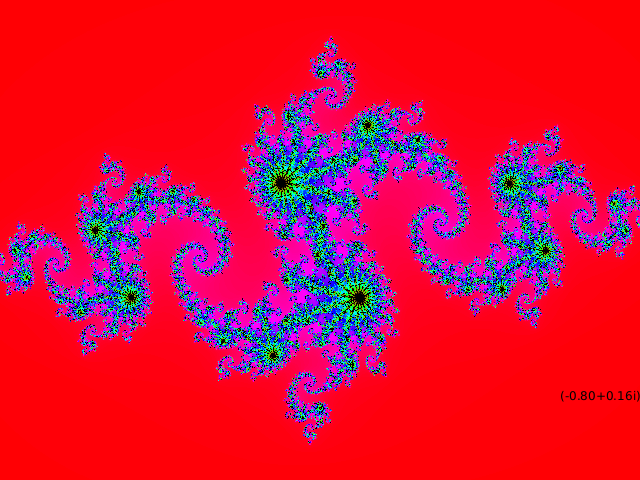I have just released a new version of propane, and found this dependant application `Julia Explorer` by Jonathon Scott (aka jscottpilgrim). Which fortunately still works with my latest release. I added the gui.

#### A video of my desktop

The video explorer inspired me to have a go at the ‘Julia Set’ challenge at Rosetta Code were I used the ruby built in Complex. I tried wrote my own Complex class to see if it would be more efficient (not much more so it seems) but it was an interesting exercise:-

``````# frozen_string_literal: true

# My Attempt to create a more efficient Complex
class MyComplex
attr_accessor :real, :imag

def initialize(real, imag)
@real = real
@imag = imag
end

# multiply complex number by another complex no
def *(other)
a = real
b = imag
c = other.real
d = other.imag
@real = a * c - b * d
@imag = a * d + b * c
self
end

# adds a given complex number
def +(other)
@real += other.real
@imag += other.imag
self
end

# computes the magnitude
def abs
Math.hypot(real, imag)
end

def to_s
format('(%<real>0.2f+%<imag>0.2fi)', real: real, imag: imag)
end
end
``````

Here’s my ‘julia sketch’ code:-

``````load_library :my_complex
CONST = MyComplex.new(-0.835, -0.2321)

def settings
size(640, 480)
end

def setup
sketch_title 'Julia Set'
@max_iter = 360
color_mode HSB, 360, 100, 100
end

def draw
grid(width, height) do |x, y|
i = max_iter
z = MyComplex.new(map1d(x, 0..width, -1.4..1.4), map1d(y, 0..height, -1.0..1.0))
while z.abs < 2 && i -= 1
z *= z
z += CONST
end
pixels[x + width * y] = color((360 * i) / max_iter, 100, i)
end
update_pixels
fill 0
text CONST.to_s, 530, 400
no_loop
end
``````

Which I used to explore some of the classic julia sets (see Wikipedia):-